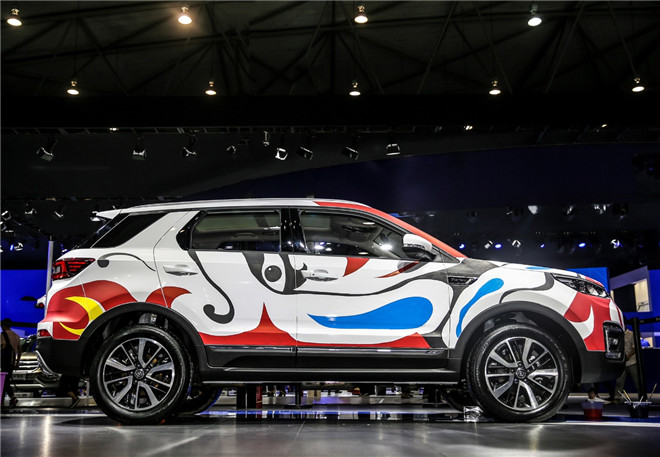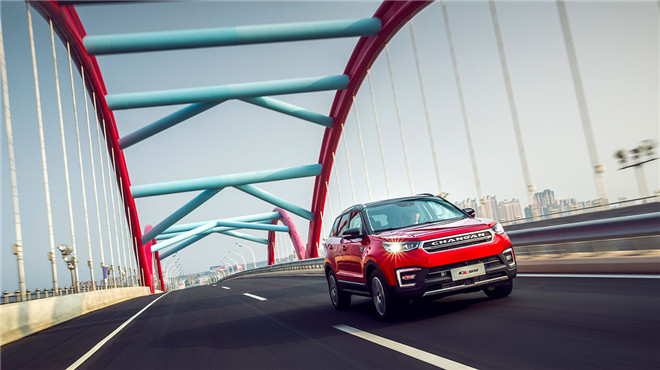## 色”名远扬的CS55 惊艳2017成都车展

2017年08月27日 18:51 来源：车行天下 超过：15316次关注

2017成都车展如火如荼的进行中，今天是公众日的第一天，吸引了众多行业人士、媒体以及观众到场参观。而长安汽车不仅上市发布了新逸动XT RS,更是让SUV CS55用绚丽的彩绘，成为了此次车展的又一热点，用色吸引住了一帮迷粉。本次车展长安汽车共携15款主流车型组成的豪华阵容亮相成都车展1号馆H104展位。为了回馈广大用户，长安汽车不仅制定了各种优惠政策，公众日期间，长安汽车还专门为喜欢长安的观众准备了丰富多彩的互动节目。其中，长安CS55彩绘作为重头戏，在公众日的第一天就以绚烂的川蜀特色主题，吸引了大家驻足，也为大家带来了不一样的视觉体验。“新轻年智色SUV”，从长安CS55的定位就不难看出，其目标客群是年轻消费者。 “新轻年”即“年纪轻、心态轻、负担轻”。 长安CS55自上海车展亮相，就引起了大家的火爆关注；上市仅12天CS55的订单就突破了1万台，横扫国产SUV市场。被公认为最“色”SUV的CS55，搭载“智色双旋”新设计理念。作为一款专为年轻消费群体打造的紧凑级SUV，CS55在吸取了长安汽车CS家族传统的设计风格的同时，又实现了设计风格新突破，运用了大胆的配色设计，而这种路线在此前长安汽车的CS家族中从未出现过，这种大胆且力求能在同级别激烈的竞争中脱颖而出。除了外观的时尚诱人，长安CS55还配备了行业领先的智能科技：其搭载Continental集成式ESC车身电子稳定控制系统，在缩短制动距离的同时，确保车辆在急刹车、急转向等突发情况下的车辆稳定状态；配备ACC全速自适应巡航系统，工作速度范围0-150km/h，可从静止激活，智能加速减速，保持安全跟车，并跟随前车停车、起步。此外，长安CS55还搭载了APA自动泊车辅助系统、PAB预警辅助制动系统、高清版360度全景影像系统、LDW车道偏离预警等智能配置，为各种技能水平的车主保驾护航。而长安CS55配备的新版in Call智能车载互联系统，集成导航、语音、娱乐、安全、远程车控等多项在线服务。长安CS55在同级SUV中空间领先。35处多变储物空间，空间利用灵活多变。在驾驶体验上，长安CS55做了深海静音级NVH处理，全车共有30余处隔吸声设计、50项风噪设计，系统化隔断噪音传播路径，配合AM3D高品质3D音效的音乐，乘坐舒适性很好。此外，优良的动力表现，搭载进口爱信新款6AT变速器，换挡平顺，配以成熟的前麦弗逊后多连杆悬架，底盘调校扎实可靠，行驶稳定性和驾驭舒适性都有不错的保障。此次车展，长安CS55不仅为观众带来了最大的视觉和触觉享受，也进一步展现长安汽车的产品实力。而除了众明星产品助阵之外，长安汽车展台更有缤纷多彩的互动环节。整个车展参观期间，您既可感受非凡的视觉体验，也可赢得惊喜礼品,还请持续关注长安汽车展台!

#### 相关文章

0-500 字已有评论 0条 查看评论>>

### 热门标签

﻿
• 快速找车
• 选择品牌
• 选择品牌
• A  奥迪
• A  阿斯顿·马丁
• A  阿尔法·罗密欧
• B  宝沃
• B  布加迪
• B  巴博斯
• B  保时捷
• B  宾利
• B  奔驰
• B  宝马
• B  本田
• B  别克
• B  标致
• B  比亚迪
• B  宝骏
• B  北汽制造
• B  北汽新能源
• B  北汽幻速
• B  北汽威旺
• B  北京汽车
• B  奔腾
• B  北汽绅宝
• C  长安
• C  长安商用
• C  长城
• C  昌河
• D  大众
• D  道奇
• D  DS
• D  东南
• D  东风风神
• D  东风风行
• D  东风小康
• D  东风风度
• D  东风
• F  福特
• F  丰田
• F  菲亚特
• F  法拉利
• F  福田
• F  福迪
• F  福汽启腾
• G  观致
• G  广汽传祺
• G  广汽吉奥
• G  GMC
• H  红旗
• H  汉腾汽车
• H  哈弗
• H  哈飞
• H  海格
• H  海马
• H  华颂
• H  黄海
• H  华泰
• H  恒天
• J  吉利汽车
• J  捷豹
• J  Jeep
• J  江淮
• J  江铃
• J  金杯
• J  九龙
• J  金旅
• K  凯翼
• K  凯迪拉克
• K  克莱斯勒
• K  科尼塞克
• K  卡威
• K  开瑞
• L  路虎
• L  林肯
• L  劳斯莱斯
• L  兰博基尼
• L  雷克萨斯
• L  铃木
• L  雷诺
• L  理念
• L  力帆
• L  莲花汽车
• L  猎豹
• L  路特斯
• L  陆风
• M  马自达
• M  MG
• M  MINI
• M  玛莎拉蒂
• M  摩根
• M  迈凯轮
• N  纳智捷
• O  欧宝
• O  讴歌
• O  欧朗
• Q  奇瑞
• Q  起亚
• Q  启辰
• R  日产
• R  荣威
• R  瑞麒
• S  三菱
• S  斯威汽车
• S  萨博
• S  smart
• S  斯柯达
• S  斯巴鲁
• S  思铭
• S  双龙
• S  上汽大通
• S  双环
• T  特斯拉
• T  腾势
• W  沃尔沃
• W  五菱汽车
• W  五十铃
• W  威兹曼
• W  威麟
• X  现代
• X  雪佛兰
• X  雪铁龙
• X  西雅特
• Y  一汽
• Y  英菲尼迪
• Y  英致
• Y  依维柯
• Y  野马汽车
• Y  永源
• Z  众泰
• Z  中华
• Z  中兴
• Z  知豆
• 选择车系
• 选择车系
• 车型对比
• 选择品牌
• 选择品牌
• A  奥迪
• A  阿斯顿·马丁
• A  阿尔法·罗密欧
• B  宝沃
• B  布加迪
• B  巴博斯
• B  保时捷
• B  宾利
• B  奔驰
• B  宝马
• B  本田
• B  别克
• B  标致
• B  比亚迪
• B  宝骏
• B  北汽制造
• B  北汽新能源
• B  北汽幻速
• B  北汽威旺
• B  北京汽车
• B  奔腾
• B  北汽绅宝
• C  长安
• C  长安商用
• C  长城
• C  昌河
• D  大众
• D  道奇
• D  DS
• D  东南
• D  东风风神
• D  东风风行
• D  东风小康
• D  东风风度
• D  东风
• F  福特
• F  丰田
• F  菲亚特
• F  法拉利
• F  福田
• F  福迪
• F  福汽启腾
• G  观致
• G  广汽传祺
• G  广汽吉奥
• G  GMC
• H  红旗
• H  汉腾汽车
• H  哈弗
• H  哈飞
• H  海格
• H  海马
• H  华颂
• H  黄海
• H  华泰
• H  恒天
• J  吉利汽车
• J  捷豹
• J  Jeep
• J  江淮
• J  江铃
• J  金杯
• J  九龙
• J  金旅
• K  凯翼
• K  凯迪拉克
• K  克莱斯勒
• K  科尼塞克
• K  卡威
• K  开瑞
• L  路虎
• L  林肯
• L  劳斯莱斯
• L  兰博基尼
• L  雷克萨斯
• L  铃木
• L  雷诺
• L  理念
• L  力帆
• L  莲花汽车
• L  猎豹
• L  路特斯
• L  陆风
• M  马自达
• M  MG
• M  MINI
• M  玛莎拉蒂
• M  摩根
• M  迈凯轮
• N  纳智捷
• O  欧宝
• O  讴歌
• O  欧朗
• Q  奇瑞
• Q  起亚
• Q  启辰
• R  日产
• R  荣威
• R  瑞麒
• S  三菱
• S  斯威汽车
• S  萨博
• S  smart
• S  斯柯达
• S  斯巴鲁
• S  思铭
• S  双龙
• S  上汽大通
• S  双环
• T  特斯拉
• T  腾势
• W  沃尔沃
• W  五菱汽车
• W  五十铃
• W  威兹曼
• W  威麟
• X  现代
• X  雪佛兰
• X  雪铁龙
• X  西雅特
• Y  一汽
• Y  英菲尼迪
• Y  英致
• Y  依维柯
• Y  野马汽车
• Y  永源
• Z  众泰
• Z  中华
• Z  中兴
• Z  知豆
• 选择车系
• 选择车系
• 选择车型
• 选择车型
• 意见反馈